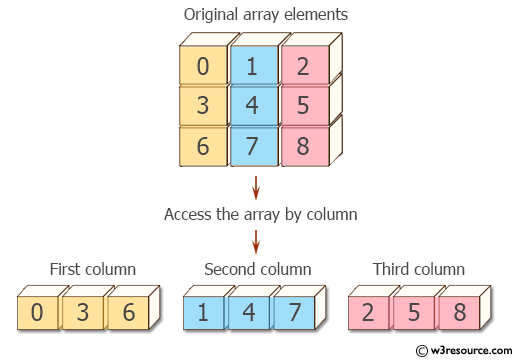﻿ NumPy: Access an array by column - w3resource# NumPy: Access an array by column

## NumPy: Array Object Exercise-81 with Solution

Write a NumPy program to access an array by column.

Pictorial Presentation:Sample Solution:

Python Code:

``````import numpy as np
x= np.arange(9).reshape(3,3)
print("Original array elements:")
print(x)
print("Access an array by column:")
print("First column:")
print(x[:,0])
print("Second column:")
print(x[:,1])
print("Third column:")
print(x[:,2])
```
```

Sample Output:

```Original array elements:
[[0 1 2]
[3 4 5]
[6 7 8]]
Access an array by column:
First column:
[0 3 6]
Second column:
[1 4 7]
Third column:
[2 5 8]
```

Python Code Editor:

Have another way to solve this solution? Contribute your code (and comments) through Disqus.

What is the difficulty level of this exercise?

Test your Python skills with w3resource's quiz

﻿

## Python: Tips of the Day

While-else construct:

```i = 5

while i > 1:
print("Whil-ing away!")
i -= 1
if i == 3:
break
else:
print("Finished up!")
```